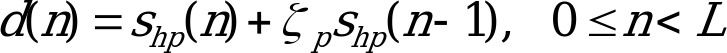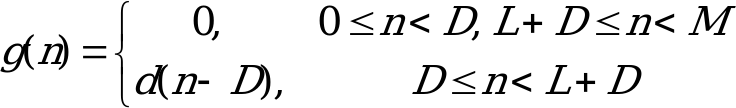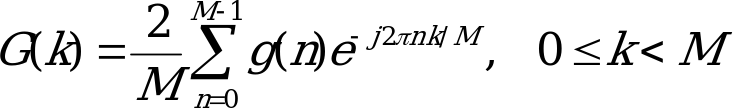## 4.3.1 Frequency Domain Conversion

06.943GPPTSVoice Activity Detector (VAD) for Adaptive Multi Rate (AMR) speech traffic channels

The input signal is pre-emphasised and windowed prior to frequency domain conversion. This process is defined as, (4.1)

where d(n) is the pre-emphasised speech buffer, p is the pre-emphasis factor, and L is the subframe length. A rectangular window is then used to frame the speech prior to frequency domain conversion, which is expressed as:, (4.2)

where D is the zero-padding offset into the DFT buffer, and M is the DFT length. The transformation of g(n) to the frequency domain is performed using the Discrete Fourier Transform (DFT) defined as:(4.3)

where ejis a unit amplitude complex phasor with instantaneous radial position .